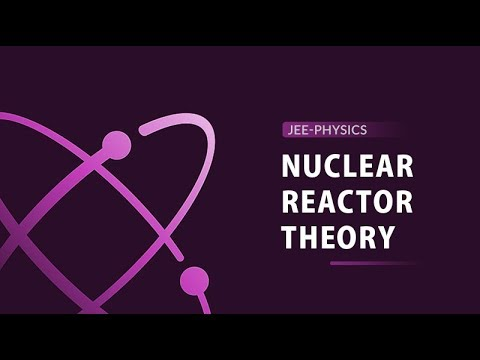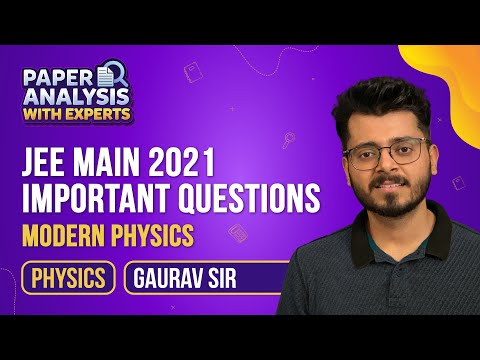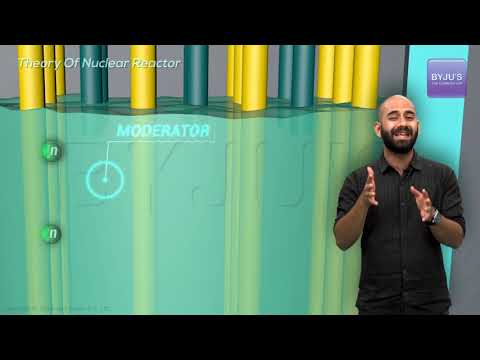Jet Set Go! All about Aeroplanes Jet Set Go! All about Aeroplanes

# Nuclear Power Plant Questions

Nuclear chemistry is the branch of chemistry that deals with the study of nuclear particles, nuclear forces and nuclear reactions. It began with the study of radioactivity. Nuclear power plants are used to generate electricity by using nuclear fission reactions. In nuclear fission reactions, splitting a heavy nucleus into two smaller fragments of approximately equal mass takes place.

 Definition: A nuclear power plant is a type of power plant used to generate electricity by using nuclear fission reactions.

## Nuclear Power Plant Chemistry Questions with Solutions

Q1. Where is Kakrapar’s atomic power station located?

(b) Gujarat

(d) None of the above

Answer: (b) Kakrapar’s atomic power station is located in Gujarat.

Q2. Where was the first nuclear power plant platnted?

(a) Bombay

(d) None of the above

Answer: (a) The first nuclear power plant was planted in Bombay.

Q3. What is the role of the moderator in the nuclear power station?

(a) Absorb the neutrons

(b) Accelerate the speed of neutrons

(c ) Reduce the speed of neutrons

(d) None of the above

Answer: (c ) The role of the moderator in the nuclear power station is to reduce the speed of neutrons.

Q4. Which of the following material is used to construct the control rod in the nuclear reactor?

(b) Copper

(c ) Graphite

(d) None of the above

Answer: (a) Cadmium is used to construct the control rod in the nuclear reactor.

Q5. Where is Narora’s atomic power station located?

(d) None of the above

Q6. What is nuclear chemistry?

Answer: Nuclear chemistry is the branch of chemistry that deals with the study of nuclear particles, nuclear forces and nuclear reactions. It began with the study of radioactivity.

Q7. Estimate the radius of 2713Al nuclei.

Ro = 1.5 X 10 -15 m

Mass Number (A) = 27

So,

r = Ro A

r = 1.5 X 10 -15 X (27)

r = 1.5 X 10 -15 X 3

r = 4.5 X 10 -15 m

r = 4.5 X 10 -15 f

Q8. What is nuclear fusion?

Answer: A reaction in which two light nuclei combine to form a heavier nucleus accompanied by the release of energy is known as nuclear fusion. It can take place by allowing highly accelerated protons and deuterons to fall on the nuclei of the lighter elements.

Q9. What is nuclear power plant?

Answer: A nuclear power plant is a type of power plant used to generate electricity by using nuclear fission reactions.

Q10. What are the primary elements of a nuclear power plant?

Answer: A nuclear power plant is a type of power plant used to generate electricity by using nuclear fission reactions. The primary elements of a nuclear power plant are mentioned below.

1. Nuclear fuel consisting of fissionable material

2. Nuclear moderator

3. Nuclear reactor coolant

4. Nuclear control rods

5. Shield or containment system

Q11. What is nuclear fission?

Answer: The splitting of a heavy nucleus into two smaller fragments of approximately equal mass is known as nuclear fission.

23592 U + 10 n → 9038 Sr + 14354 Xe + 3 10 n

Q12. What are alpha beta and gamma rays?

Answer: Alpha rays: The rays deflected towards the negative plate and positively charged are known as alpha rays. These rays consist of merely helium nuclei.

Beta rays: The rays deflected toward the positive plate and are negatively charged are known as beta rays. These rays consist of mere electrons.

Gamma rays: The rays which are not deflected at all and are neutral are known as gamma rays.

Q13. The ratio of the mass of Pb206 to the mass of U238 in a certain rock specimen is 0.5. Assuming that the original rock contains no lead, estimate its age. Given the half-life of uranium is 4.5 X 109 years.

We know that

U238 = (U238 + Pb206) e -𝜆t

Or

1 = (1 + Pb206 / U238 ) e -𝜆t

1 = (1 + 0.5) e -𝜆t

1 = 1.5 e -𝜆t

e 𝜆t = 1.5

𝜆t = 2.3 log10 1.5

𝜆t = 2.3 X 0.1761

(0.693 / 4.5 X 109) X t = 0.23 X 0.1761

t = 2.63 X 109 years.

Q14. Match the following.

Column A

Column B

Nuclear fusion

Fission and fusion

Fission in a nuclear reactor

6027 Co nucleus

β Decay

Absorption of thermal neutrons by 23592 U

γ ray emission

Energy production in stars via hydrogen conversion to helium

Column A

Column B

Nuclear fusion

Energy production in stars via hydrogen conversion to helium

Fission in a nuclear reactor

Absorption of thermal neutrons by 23592 U

β Decay

6027 Co nucleus

γ ray emission

Fission and fusion

Q15. How will you differentiate between nuclear fusion and nuclear fission?

Answer: We can distinguish between nuclear fusion and fission in the following ways.

S. No.

Nuclear Fusion

Nuclear Fission

1.

Nuclear fusion is defined as the combining of two lighter nuclei into a heavier one.

Nuclear fission is defined as the splitting of a nucleus into two daughter nuclei

2.

The energy released in the nuclear fusion reaction is much more than in the nuclear fission reaction.

A lot of energy is released in nuclear fission.

3.

Fusion reactions occur in stars and the sun.

Fission reactions do not occur naturally

4.

A large amount of energy is supplied in the nuclear fusion reaction.

Little energy is supplied in the nuclear fission reaction.

5.

Hydrogen bomb works on the principle of a nuclear fusion bomb.

The atomic bomb works on the principle of nuclear fission

6.

Hydrogen isotopes are the primary fuel used in power plants.

Uranium is the primary fuel used in power plants

## Practise Questions on Nuclear Power Plant

Q2. What is the significance of the nuclear study?

Q3. Disseminate the reaction of the splitting of Plutonium-239 in a nuclear fission reaction.

Q4. Disseminate the reaction of the splitting of Uranium-233 in a nuclear fission reaction.

Q5. What happens when neutrons are bombarded on the uranium-235 atom?

Click the PDF to check the answers for Practice Questions.

## Theory of Nuclear Reactor## Top 15 Most Important and Expected Questions on Nuclei## Modern Physics Class 11 & 12## Theory of Nuclear Reactor